Test: Buoyancy & Floatation - 2

# Test: Buoyancy & Floatation - 2

Test Description

## 10 Questions MCQ Test Fluid Mechanics | Test: Buoyancy & Floatation - 2

Test: Buoyancy & Floatation - 2 for Mechanical Engineering 2022 is part of Fluid Mechanics preparation. The Test: Buoyancy & Floatation - 2 questions and answers have been prepared according to the Mechanical Engineering exam syllabus.The Test: Buoyancy & Floatation - 2 MCQs are made for Mechanical Engineering 2022 Exam. Find important definitions, questions, notes, meanings, examples, exercises, MCQs and online tests for Test: Buoyancy & Floatation - 2 below.
Solutions of Test: Buoyancy & Floatation - 2 questions in English are available as part of our Fluid Mechanics for Mechanical Engineering & Test: Buoyancy & Floatation - 2 solutions in Hindi for Fluid Mechanics course. Download more important topics, notes, lectures and mock test series for Mechanical Engineering Exam by signing up for free. Attempt Test: Buoyancy & Floatation - 2 | 10 questions in 30 minutes | Mock test for Mechanical Engineering preparation | Free important questions MCQ to study Fluid Mechanics for Mechanical Engineering Exam | Download free PDF with solutions
 1 Crore+ students have signed up on EduRev. Have you?
Test: Buoyancy & Floatation - 2 - Question 1

### When a body, floating in a liquid is given a small angular displacement, it starts oscillating about a point known as

Detailed Solution for Test: Buoyancy & Floatation - 2 - Question 1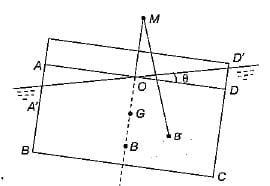Where M is metacentre and θ is small angle of hill through which a body is tilted.
Hence metacenter is a point about which a body oscillate when tilted or given a small angular displacement.

Test: Buoyancy & Floatation - 2 - Question 2

### The time of oscillation of a floating body is given by Where K = Radius of Gyration of the floating body about its centre of gravity h = Metacentric height of the floating body

Detailed Solution for Test: Buoyancy & Floatation - 2 - Question 2

Time of oscillation of a floating body is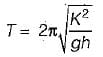h = metracentric height

Test: Buoyancy & Floatation - 2 - Question 3

### The time of oscillation of a floating body with increase in metacentric height will be

Detailed Solution for Test: Buoyancy & Floatation - 2 - Question 3

Time of oscillation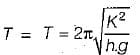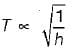as h increases, time of oscillation decreases

Test: Buoyancy & Floatation - 2 - Question 4

A floating body is in stable equilibrium when

Detailed Solution for Test: Buoyancy & Floatation - 2 - Question 4

For stable equilibrium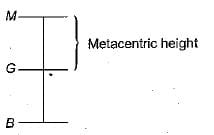Test: Buoyancy & Floatation - 2 - Question 5

A submerged body will be in stable equillibrium. if

Detailed Solution for Test: Buoyancy & Floatation - 2 - Question 5

For stable equilibrium of submerged body: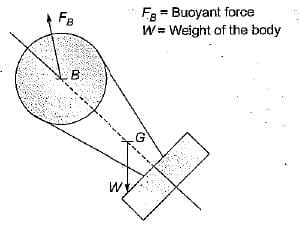Hence for stable equilibrium of submerged body centre of buoyancy B should be above G.

Test: Buoyancy & Floatation - 2 - Question 6

Which one of the following is the conditions for stable equilibrium for a floating body

Detailed Solution for Test: Buoyancy & Floatation - 2 - Question 6

For stable equilibrium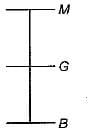For stable equilibrium metacenter is above the center of gravity.

Test: Buoyancy & Floatation - 2 - Question 7

How is the metacentric height (GM) expressed
Where I = Moment of inertia of the plan of the floating body at the water surface
V = Volume of the body submerged in water
BG = Distance between the center of gravity (G) and the center of Buoyancy (B)

Detailed Solution for Test: Buoyancy & Floatation - 2 - Question 7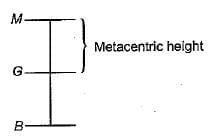Metacentric height,
GM = BM - BG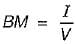Where I = Area moment of inertia of top view about longitudinal axis
V = Volume of the fluid displaced
Hence,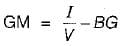Test: Buoyancy & Floatation - 2 - Question 8

An odd shaped body weighing 7.5 kg and occupying 0.01 m3 volume wiil be completely submerged in a fluid having specific gravity of

Test: Buoyancy & Floatation - 2 - Question 9

A cylindrical body of cross-sectional area A height H and density ρs is immersed to a depth h in a liquid of density ρ and tied down to bottom with a string then the tension in the string is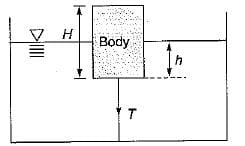Detailed Solution for Test: Buoyancy & Floatation - 2 - Question 9

w + T =  FB = weight of fluid displaced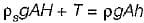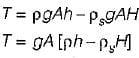Test: Buoyancy & Floatation - 2 - Question 10

A float valve of the ‘ball-cock’ type required to close an opening of a supply pipe feeding a cistern as shown in the given figure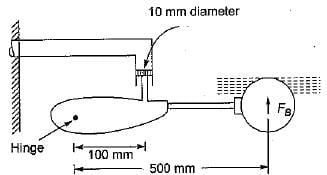The buoyant force FB required to be exerted by the float to keep the valve closed against a pressure of 0.28 N/mm2 is

Detailed Solution for Test: Buoyancy & Floatation - 2 - Question 10

Taking moment about hinge We get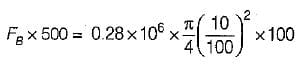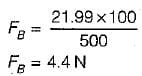## Fluid Mechanics

43 videos|72 docs|72 tests
 Use Code STAYHOME200 and get INR 200 additional OFF Use Coupon Code
Information about Test: Buoyancy & Floatation - 2 Page
In this test you can find the Exam questions for Test: Buoyancy & Floatation - 2 solved & explained in the simplest way possible. Besides giving Questions and answers for Test: Buoyancy & Floatation - 2, EduRev gives you an ample number of Online tests for practice

## Fluid Mechanics

43 videos|72 docs|72 tests